# What is an Absolute Reference in Excel

In many of my articles, you must have read word absolute reference. If not, you must have notices \$ (dollar) sign before cell references (\$A\$1). They are absolute references. When we prefix the \$ sign before a column letter and/or row number that row and/or column number becomes absolute.What does excel absolute reference mean?

Well, when we don’t want cell references to change relatively while copying them in other cells, we use absolute references in excel. In other words, we lock or say freeze the cell or range using absolute referencing.
Generic Syntax of Absolute Referencing

Syntax to Freeze column only (Absolute Column Reference)

=\$A1

Syntax to Freeze Row Only (Absolute Row Reference)

=A\$1

Syntax of Freeze Cell (Absolute Cell Reference)

=\$A\$1

Syntax of Expanding Range (Absolute Starting Cell Reference)

=\$A\$1:A1

Syntax of Collapsing Range (Absolute Ending Cell Reference)

=A1:\$A\$10

Syntax for Frozen Range (Absolute Starting Cell Reference)

=\$A\$1:\$A\$10

Let’s see an example for each to make things clear.

Example: Excel Absolute Cell Reference
So to illustrate the use of all above types of Absolute referencing, I have this 2D matrix in excel.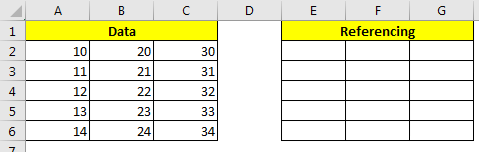In range A2:C6 I have some numbers. In range E2:G6 we will test above absolute referencing techniques of excel.

The shortcut to make absolute references is F4 while selecting the range in formula.

Absolute Column Only (Freeze Column)

When we only want to freeze column only, we put \$ sign before the column latter of cell. This is also called mixed referencing; since rows are relative and column is absolute.
Let’s se the effect of it.

In E2, write this formula and hit enter.

=\$A2

Now you can see the value of A2 in E2. Copy E2 in rest of the cells in range E2:G6. What you see?
You can see that the only A columns data is being copied in all cell in E2:G6. When you copy down the rows are changing. When you copy right ward or left ward, columns are not changing. This is because we frozeon the column.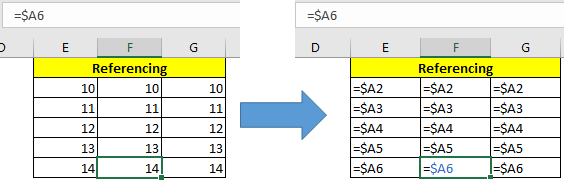Absolute Rows Only (Freeze Rows)
It same as freezing columns. We use \$ sign before row number and leave column number relative. This makes excel to freeze the row and keep columns relative.

Write this formula in E2 and copy E2 in rest of the range.

=A\$2

You will see that, only row 2’s data is copied in all the rows since row 2 is frozen using \$ sign.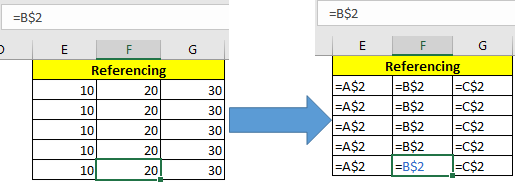Syntax of Freeze Cell Only (Absolute Cell Reference)

When we use \$ sign before both, column letter and row number, this become absolute cell reference in excel. It means when you copy formula, the formula will always refer to frozen cell.

Write this formula in E2 and copy E2 in rest of the range.

=\$A\$2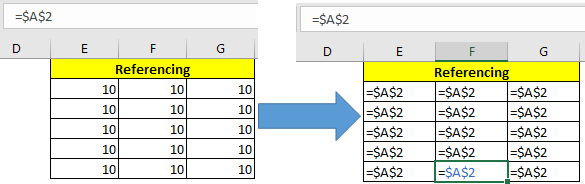Expanding Range (Absolute Starting Cell Reference)

When we reference a range and make the first cell absolute, this range becomes an expanding range. When we copy such formula, downwards or rightwards, the range expands.

Write this sum formula in E2 and copy E2 downwards and to right of excel sheet.

=SUM(\$A\$2:A2)

You’ll see that the first cell E2 has value A2 only. E3 cell has sum of A2:A3 and E6 has sum of A2:A6. Similar thing happens to the right of sheet.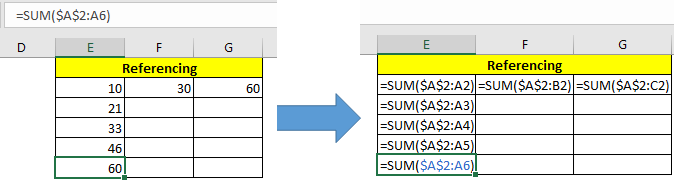Collapsing Range (Absolute Ending Cell Reference)

To apposite of above technique, this type of reference collapses when you copy downwards.

Write this sum formula in E2 and copy E2 downwards.

=SUM(A2:\$A\$6)

You can see that first cell contains the sum of of A2:A6, next A3:A6 and last cell contains A6:A6.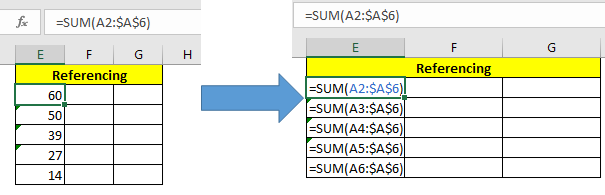Absolute Ranges in Excel
This is one of most used type of absolute referencing. Here we make the whole range absolute.
Write this formula in cell E2 and copy E2 in other cells.

=SUM(\$A\$2:\$A\$6)

You can see that all the cells have sum of A2:A6.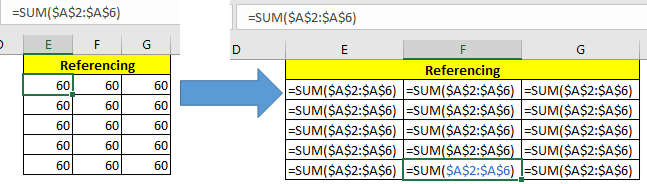Most of the people make mistake while using lookup formulas by giving relative references of lookup range. You should always give absolute references of lookup range while using any lookup formula. This will make sure that lookup range doesn’t change (until you deliberately want it) while you copy formula in other cells.

Eg =VLOOKUP (E2,\$A\$2:\$A\$6,1,0).
So yeah guys, this how we make absolute range in excel and use it in different ways to achieve our objective. There are many other ways to use \$ sign. Explore them your selves and let me know in the comments section. If you have any excel trick or tip under your sleeves, then share it to world. Right down in the comments section below. Feel free to ask any question.
Related Articles:

Expanding References in Excel

Relative and Absolute Reference in Excel

Shortcut To Toggle Between Absolute and Relative References in Excel

Dynamic Worksheet Reference

All About Named Ranges In Excel

Total number of rows in range in excel

Dynamic Named Ranges in Excel

Popular Articles:

The VLOOKUP Function in Excel

COUNTIF in Excel 2016

How to Use SUMIF Function in Excel

Terms and Conditions of use

The applications/code on this site are distributed as is and without warranties or liability. In no event shall the owner of the copyrights, or the authors of the applications/code be liable for any loss of profit, any problems or any damage resulting from the use or evaluation of the applications/code.